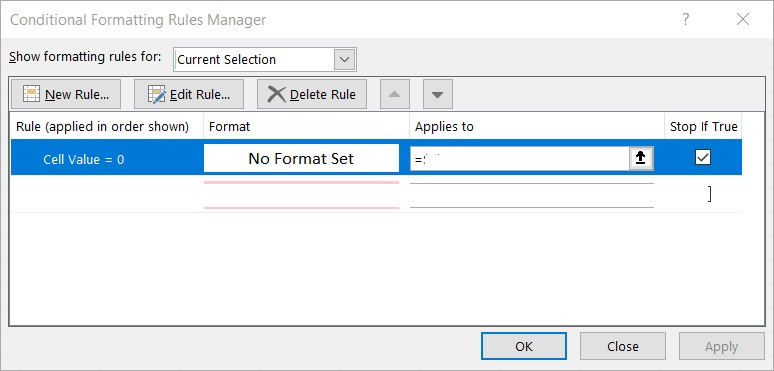# Rookie Excel formula question

Hi I would like to use conditional formatting so that that if the result of a caculation is positive zero or negative then background colour the cell appropriately.  The issue is, if the cells being used are blank then I want the result to be blank (rather than zero), so that no formatting applies.

This is what I have at the moment:
=IF(AND(ISBLANK(E6),ISBLANK(G6)),"",E6-G6)
To try and get a blank target cell when E6 and G6 are blank, this seems rather ineffiecent, is there a better way of doing it?

The same with summation
=SUMIF(E6:E17,"<>""") still results in a '0' in the target cell how can I make the result blank if the range E6:E17 is blank?

Microsoft ExcelMicrosoft OfficeLast Comment
Subodh Tiwari (Neeraj)NatchiketSeems I have to use something like this:
=IF(SUMPRODUCT(--(E6:E7<>""))=0,"",SUM(E6:E7))
Again, is there a better way ?Subodh Tiwari (Neeraj)One way is this...

If you want the SUM formula to sum the range E6:E17 if no cell in this range is empty, you may try the following formula...
``````=IF(COUNTBLANK(E6:E17)=ROWS(E6:E17),"",SUM(E6:E17))
``````

and then you can use the below formula to make a new rule for conditional formatting for the formula cell.
e.g. if you formula cell is F6, the conditional formatting formula for highlighting the cell if the sum returned in F6 is greater than 0 would be...
``````=AND(ISNUMBER(F6),F6>0)
``````
Same way you can construct the formula for other conditional formatting rules.Ryan ChongWhy not simply create a new rule with "No Format Set" and put at the top of your rules?

make sure you ticked the "Stop if True" option for this rule too.NatchiketCurrently I am having this problem
Cell M6 has the formula: =IF(AND(ISBLANK(G6),ISBLANK(I6)),"",G6-I6) so it is blank if G6 and I6 are blank
But cell BE6 has the formula =M6+W6+AG6+AQ6+BA6   but the "" in M6 causes the expression #VALUE to appear in BE6Subodh Tiwari (Neeraj)THIS SOLUTION IS ONLY AVAILABLE TO MEMBERS.
View this solution by signing up for a free trial.
Members can start a 7-Day free trial and enjoy unlimited access to the platform.Microsoft Excel

Microsoft Excel topics include formulas, formatting, VBA macros and user-defined functions, and everything else related to the spreadsheet user interface, including error messages.

144K
Questions
--
Followers
--
Top Experts
Get a personalized solution from industry experts

TRUSTED BY